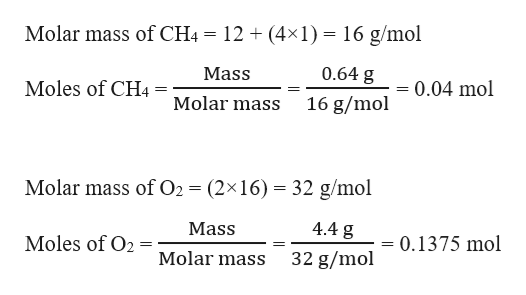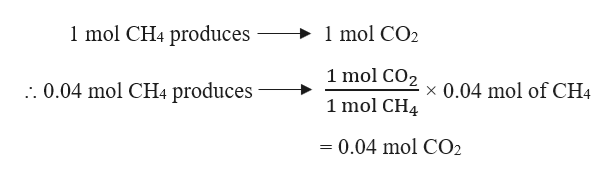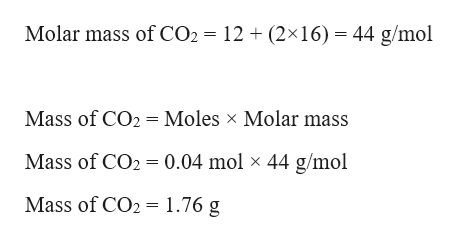# Gaseous methane (CH,) reacts with gaseous oxygen gas (0,) to produce gaseous carbondioxide (co,) and gaseous water (H,0). If 1.07 g of carbon dioxide is produced from the reactionof 0.64 g of methane and 4.4 g of oxygen gas, calculate the percent yield of carbon dioxide.Round your answer to 2 significant figures.aloExplanationCheck© 2019 McGraw-Hill Education. All Rights Reserved. Terms of Use | Privacy

Question
3 views
check_circle

Step 1

Given,

Mass of CH4 = 0.64 g

Mass of O2 = 4.4 g

Mass of CO2 produced = 1.07 g

Moles of CH4 and Br2 can be calculated as:help_outlineImage TranscriptioncloseMolar mass of CH4 = 12 + (4×1) = 16 g/mol 0.64 g Mass = 0.04 mol Moles of CH4 Molar mass 16 g/mol Molar mass of O2 = (2×16) = 32 g/mol 4.4 g Mass Moles of O2 0.1375 mol Molar mass 32 g/mol fullscreen
Step 2

The balanced chemical reaction of gaseous methane and oxygen gas to produce gaseous carbon dioxide and gaseous water can be written as:

CH4 (g) + 2 O2 (g) → CO2 (g) + 2 H2O (g)

Stoichiometric molar ratio of CH4 : O2 = 1 : 2

Actual molar ratio of CH4 : O2 = 0.04 : 0.1375 = 1 : 3.4

Therefore, O2 is present in excess and CH4 is the limiting reagent. Therefore, the theoretical moles of CO2 produced will depend on CH4.

From the given reaction, it is evident that :help_outlineImage Transcriptionclose1 mol CH4 produces 1 mol CO2 1 mol CO2 1 mol CH4 :. 0.04 mol CH4 produces x 0.04 mol of CH4 = 0.04 mol CO2 fullscreen
Step 3

Theoretical mass of CO2 produced c...help_outlineImage TranscriptioncloseMolar mass of CO2 = 12 + (2×16) = 44 g/mol Mass of CO2 = Moles x Molar mass Mass of CO2 = 0.04 mol × 44 g/mol Mass of CO2 = 1.76 g fullscreen

### Want to see the full answer?

See Solution

#### Want to see this answer and more?

Solutions are written by subject experts who are available 24/7. Questions are typically answered within 1 hour.*

See Solution
*Response times may vary by subject and question.
Tagged in

### Chemistry